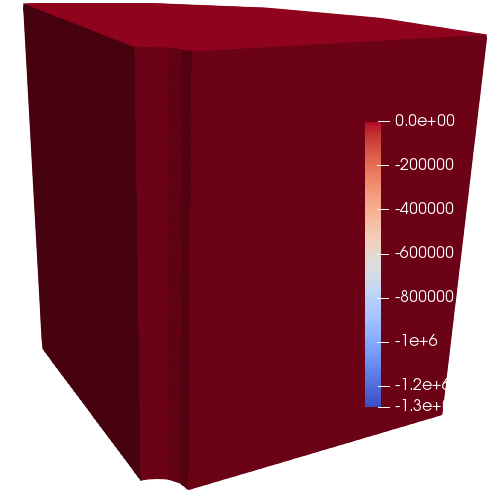# Porous Flow Tutorial Page 08. The PorousFlowSink and unsaturated flow

The PorousFlowSink has been mentioned in this tutorial already, but now is an appropriate time to study it more carefully. It is a very powerful BC which can handle virtually all physical situations. It also helps enormously in resolving some convergence problems. Therefore, it is well worth studying, so please feel free to take a break from this tutorial and read the info here.

Now that you've finished studying the PorousFlowSink let's apply it in a simple situation. The purely fluid-flow model of Page 01 is extended to include unsaturated flow. Firstly, the PorousFlowBasicTHM Action must be replaced by a PorousFlowUnsaturated Action:

[PorousFlowUnsaturated]
porepressure = porepressure
coupling_type = Hydro
gravity = '0 0 0'
fp = the_simple_fluid
relative_permeability_exponent = 3
relative_permeability_type = Corey
residual_saturation = 0.1
van_genuchten_alpha = 1E-6
van_genuchten_m = 0.6
[]

(modules/porous_flow/examples/tutorial/08.i)

There are evidently some more input parameters:

• The relative_permeability parameters. These are documented in Relative permeability

• The van_genuchten capillarity parameters. These are documented in Capillary pressure

The PorousFlowConstantBiotModulus is not needed here (it's only needed by PorousFlowBasicTHM). Because of the multiplication of the fluid mass-balance equation by density, an appropriate nl_abs_tol is in contrast to the calculated on Page 02:

[Executioner]
type = Transient
solve_type = Newton
end_time = 1E6
dt = 1E5
nl_abs_tol = 1E-7
[]

(modules/porous_flow/examples/tutorial/08.i)

Finally we get to the PorousFlowSink. In the following, fluid is extracted through injection_area at a constant rate of kg.s.m. However, in the unsaturated region () this rate is modified by the relative permeability because use_relperm = true. This greatly improves convergence and in many cases is physically reasonable as there are limits to pumps and other similar machinery.

[BCs]
[./production]
type = PorousFlowSink
variable = porepressure
fluid_phase = 0
flux_function = 1E-2
use_relperm = true
boundary = injection_area
[../]
[]

(modules/porous_flow/examples/tutorial/08.i)

An animation of the results is shown in Figure 1.Figure 1: Porepressure evolution in the production version of the borehole-aquifer-caprock system.

Try to run the simulation with use_relperm = false and you'll see very poor convergence. The total fluid extracted is kg (the product of rate, area and total time), while the model contains kg (the product of porosity, fluid density, and model volume), so the model is not running completely dry, but it requires extremely low porepressures to affect the constant rate of fluid removal ( gets so low that MOOSE starts to suffer from precision loss).

The simulation may be promoted to a full THMC simulation using the approach used in Page 03, Page 04 and Page 06. The arguments made about scaling the variables must be modified to take into account the fluid density appearing in the fluid equation (see Page 06) so the scaling will be approximately for the temperature and for the displacement variables. The porosity may depend on porepressure, temperature, volumetric strain and chemistry.

The simulation described so far uses full upwinding, which is the default in PorousFlow. TVD stabilization (see also numerical diffusion and a worked example of KT stablization) may be used instead by simply changing the PorousFlowUnsaturated block to:

[PorousFlowUnsaturated]
porepressure = porepressure
coupling_type = Hydro
gravity = '0 0 0'
fp = the_simple_fluid
relative_permeability_exponent = 3
relative_permeability_type = Corey
residual_saturation = 0.1
van_genuchten_alpha = 1E-6
van_genuchten_m = 0.6
stabilization = KT
flux_limiter_type = None
[]

(modules/porous_flow/examples/tutorial/08_KT.i)